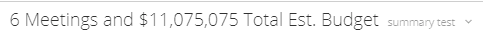# Help on Beast Mode Concat Format

Current I get for a Summary 2711 Meetings and \$143043062 Total Est. Budget but I am looking for the \$ amount to include comma separators and two decimal. Am using a beast mode of CONCAT(COUNT(`Meeting Identifier`),' Meetings and \$', SUM(`Total Estimated Budget`),' Total Est. Budget') and I am a novice and painfully stuck. Any help is apprecaited.

• What we could really use here is the FORMAT() function.  @DaniBoy  (hint, hint)

However, as that is not an option for beastmodes, we will have to get more creative with our solution.

It's going to look something like this... but I have not had the time to test it out:

`CONCAT(  COUNT(`Meeting Identifier`)  ,' Meetings and \$'  ,left(   round(sum(`Total Estimated Budget`),2),   MOD(      (LENGTH(round(sum(`Total Estimated Budget`),2))),3      ))   ,case when (LENGTH(round(sum(`Total Estimated Budget`),2))/3) >1 then ',' else '.' end  ,case when (LENGTH(round(sum(`Total Estimated Budget`),2))/3) >1 then SUBSTRING(round(sum(`Total Estimated Budget`),2),MOD(      (LENGTH(round(sum(`Total Estimated Budget`),2))),3      )+1,3) else RIGHT(round(SUM(`Total Estimated Budget`),2),2) end  ,case when (LENGTH(round(sum(`Total Estimated Budget`),2))/3) >2 then ',' end  ,case when (LENGTH(round(sum(`Total Estimated Budget`),2))/3) >2 then SUBSTRING(round(sum(`Total Estimated Budget`),2),MOD(      (LENGTH(round(sum(`Total Estimated Budget`),2))),3      )+1,3) else RIGHT(round(SUM(`Total Estimated Budget`),2),2) end    ,' Total Est. Budget'   )`

I did get this to work with my sample data:______________________________________________________________________________________________
“There is a superhero in all of us, we just need the courage to put on the cape.” -Superman
______________________________________________________________________________________________